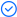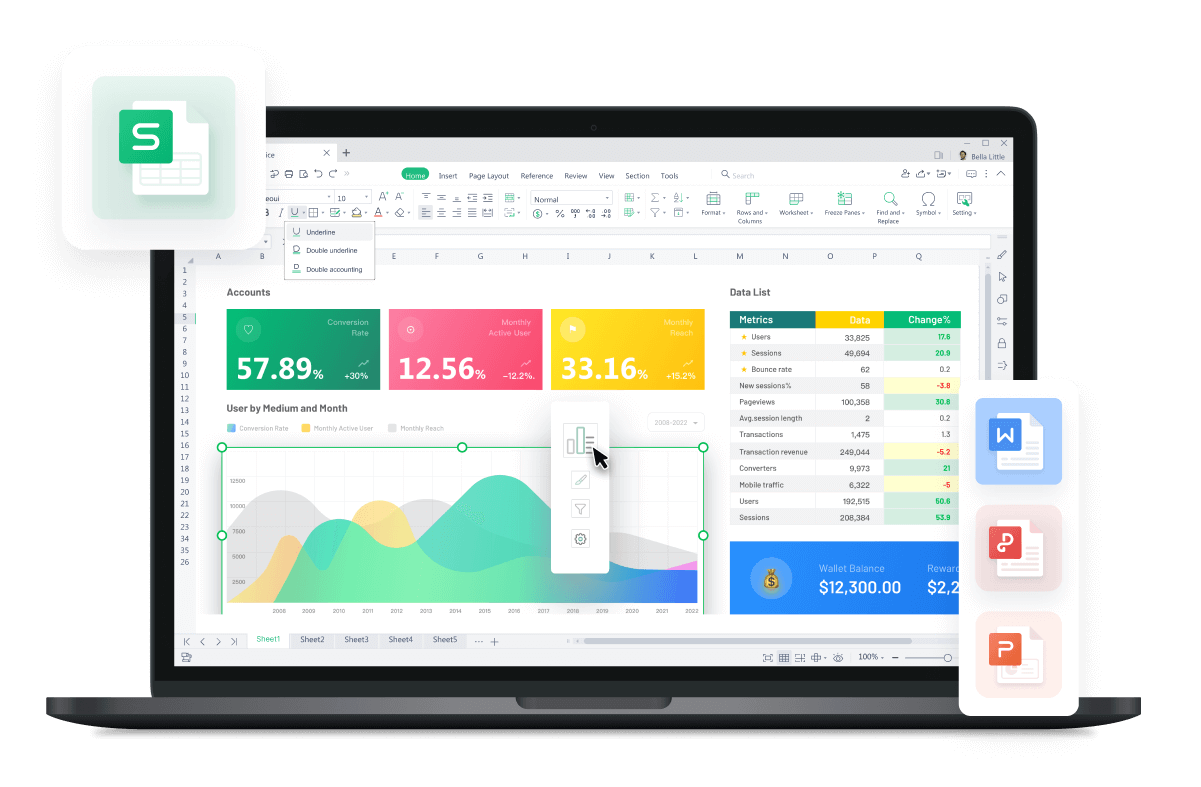WPS Office

Free All-in-One Office Suite with PDF EditorRead, edit, and convert PDFs with the powerful PDF toolkit.Microsoft-like interface, easy to use.

Windows • MacOS • Linux • iOS • Android# How to use IF, AND, OR functions in excel with text

August 1, 2022
3.1K Views

Learning how to use IF, AND, OR functions in excel with text could be quite useful. This article is going to show you how to IF, AND, OR functions in excel with text. The IF function is used to return one value if a condition is met, or another value if it isn't. It takes three arguments: a logical test and two values that are returned if the statement is true or false respectively. The AND and OR functions take an array of values as their arguments. They return TRUE if all the values in their argument list evaluate to TRUE, or FALSE otherwise. In this tutorial, we'll walk through how to IF, AND, OR functions in excel with text so that you can get started making decisions in your own spreadsheets today!

How to use IF function in excel?

We will show you how to use IF, AND, OR functions in excel with text but we will start with the IF function. The IF function is one of the most powerful and useful functions in Excel. It can be used to create an array of logical decisions and conditions, which can help you get more out of your spreadsheets.

This method works best with versions 2016/2016/mac/online.

1. Excel does not recognize case sensitivity when using the IF function. This means that for text values, the IF formula will return the same result regardless of whether or not you capitalize or use lowercase letters in your formulas.

2. You can follow the given syntax when using IF function:

=IF(logic test,value if true,value if false)

How to use AND function in excel?

Excel is an incredibly powerful tool, and the AND function is one of the most useful mathematical tools you can use to make your work easier. This guide will help you understand how to use the AND function in Excel with text.

This method works best with versions 2016/2016/mac/online.

1. The Excel AND function, an example of a logical function, requires more than one condition to be met at the same time. The function returns either TRUE or FALSE.

2. You can follow the given syntax when using IF function:

=AND (logical1, logical2, ...)

How to use OR function in excel?

OR function in excel is one of the most useful functions. The Excel OR function is used for finding the intersection of two sets of data. You can use it to compare two values and test whether a relationship exists between them.

This method works best with versions 2016/2016/mac/online.

1. The OR function is used to return TRUE if any of two or more arguments are TRUE. It returns FALSE if all arguments are FALSE.

2. You can follow the given syntax when using IF function:

=OR (logical1, logical2, …)

Did you learn about how to use IF, AND, OR functions in excel with text? Do you now know how to use IF, AND, OR functions in excel with text? You can follow WPS Academy to learn more features of Word Document, Excel Spreadsheets and PowerPoint Slides.